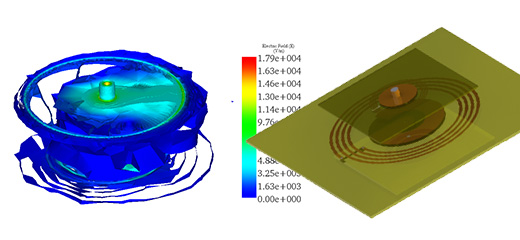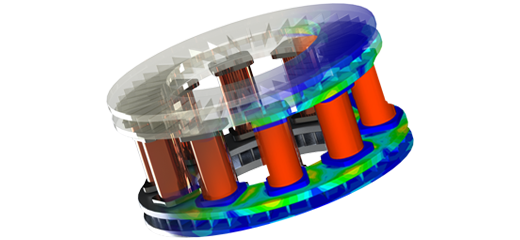RF and Thermal Simulation of Straight Ridge Waveguide Coupler

Passive components Electro-thermal RF & Microwave Components Electronic Design Automation & Electronics Multiphysics

Introduction

Rectangular waveguides of WR2300 half height type are widely used for coupling power to accelerate cavity through an iris between the end ridge and the accelerator cavity. The present study focuses, however, on the transition part only as the iris coupling is adjusted depending on the type of cavity and coupling requirements. The parameters of interest in high power accelerators systems are returns loss, insertion loss, resonant frequency, and its thermal behavior.

This study illustrates the design and simulation features using the duo Solidworks and HFWorks to enable users to envision and design RF couplers. A coupled RF to thermal analysis of the waveguide is presented. The S-Parameters module of HFWorks with coupling to thermal is used to simulate this waveguide.

Design and Simulation

Figure1, shows the coupler structure designed with Solidworks. This structure consists of three parts. An incoming rectangular WR2300 half height waveguide named part I, a central ridge waveguide named part II, and an end ridge named part III, as shown in Figure2. The overall size of the straight ridge waveguide coupler is 400mm x 400mm x 150mm.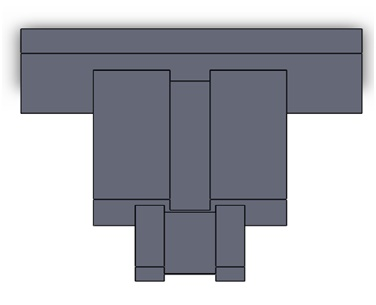Figure 1 - The 3D Solidworks model of straight ridge waveguide coupler

The schematic diagram of the side view of the waveguide coupler is shown in Figure2. The variation of the cut off frequency as a function of the ridge gap for TE10 mode is simulated using ATLASS  and shown in Figure3.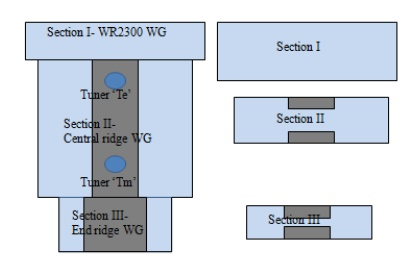Figure 2 - The schematic diagram of the waveguide coupler

The geometrical parameters can be defined in a file and imported into Solidworks using the Equations feature of the software. These parameters are summarized in Table 1.

Table 1 - Dimensional details of the coupler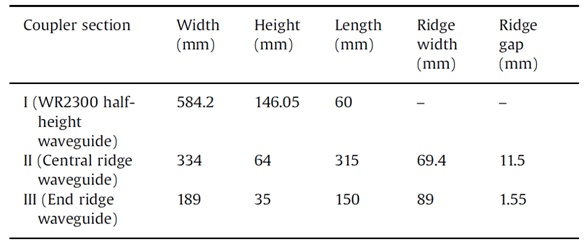Results

Figure3, shows the variation of the cut off frequency for TE10 mode using ATLASS, by varying the ridge gap.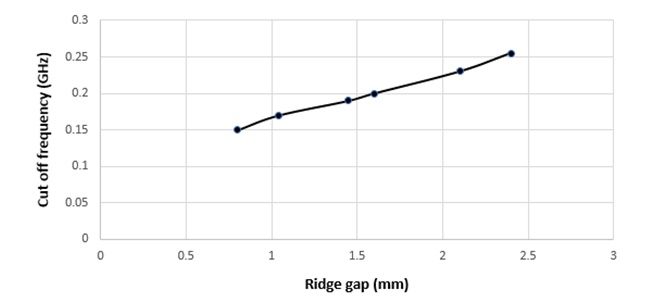Figure 3 - Cut off frequency in function of ridge gap

The simulated return loss S11 and insertion loss S21 using HFWorks and the measured results of the coupler are depicted in Figure4.  The waveguide coupler resonates at 0.353 GHz, as shown in Figure4.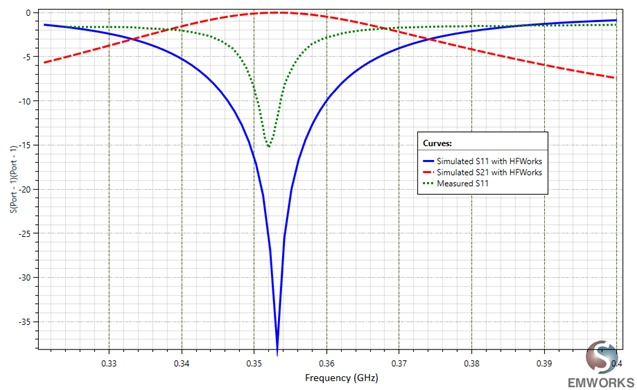Figure 4 - Return and insertion losses of the straight ridge waveguide coupler

HFWorks automatically computes the conductor loss, i.e. the thermal load, on the coupler walls and feeds it into the thermal module assuming an incident power of 250kW.  The surrounding air is assumed to have a connective heat transfer coefficient (h) of  1000W / m2 and an ambient temperature of 293 K.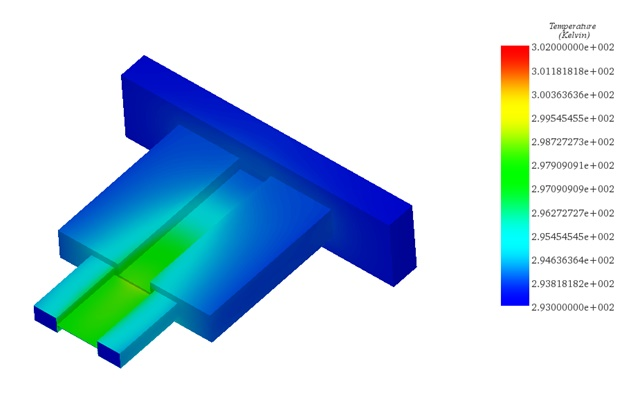Figure 5 - The temperature distribution at 0.353 GHz and an incident power of 250kW

The distribution of the temperature is shown in Figure5.  Clearly, there is little increase in the temperature despite the incident power of 250kW which assures the absence of any thermos-structural defect on the waveguide.

Conclusion

An RF straight ridge waveguide coupler for coupling power to accelerators is simulated using HFWorks. The insertion loss, return loss, and the resonant frequency are presented and compared to measured data  showing a good agreement. Unfortunately, the reference does not have any thermal simulation. Therefore, we present the thermal results without comparing to any reference.

References

 https://www.emworks.com/product/ATLASS  Rajesh Kumar, Mentes Jose, G.N. Singh, Girish Kumar and P.V. Bhagwat "RF characterization and testing of ridge waveguide transitions for RF power couplers ", published at Nuclear Instruments and Methods in Physics Research A 838, pp. 66-7, 2016

Upcoming webinar